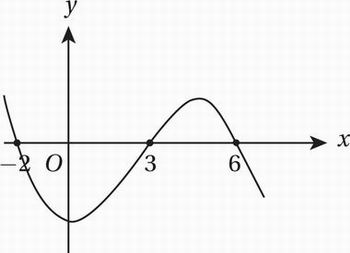# SAT Math Multiple Choice Question 615: Answer and Explanation

### Test Information

Question: 615

15. The function g(x) = ax3 + bx2 + cx + d has zeroes at x = -2, x = 3, and x = 6. If g(0) < 0, which of the following must also be negative?

• A. g(-3)
• B. g(-1)
• C. g(4)
• D. g(5)